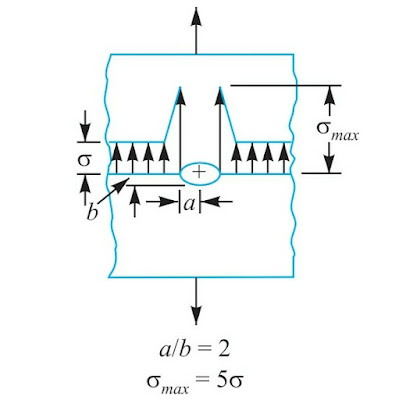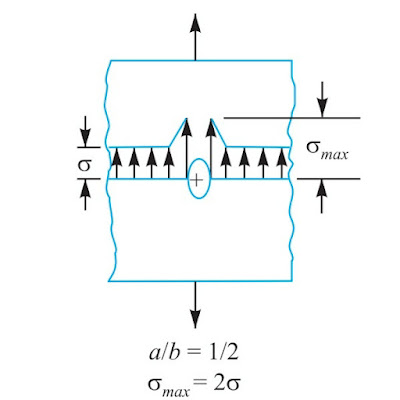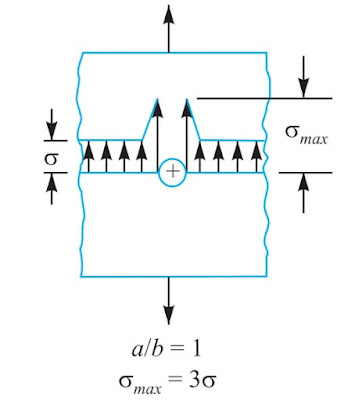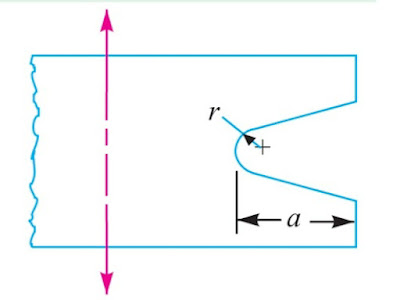# Stress Concentration due to Holes and Notches

Stress Concentration due to Holes and Notches,
Consider a plate with a transverse elliptical hole as shown in Figure (a) and is subject to tensile load. We see from the stress-distribution that the stress at the point away from the hole is practically uniform and the maximum stress will be induced at the edge of the hole. Maximum stress is given by
$$\sigma_{\max }=\sigma\left(1+\frac{2 a}{b}\right)$$
and the theoretical stress concentration factor,
$$K_{t}=\frac{\sigma_{\max }}{\sigma}=\left(1+\frac{2 a}{b}\right)$$
When a/b is large, the ellipse approaches a crack transverse to the load and the value of$$K_{t}$$becomes very large. When a/b is small, the ellipse approaches a longitudinal slit [as shown in Figure (b)] and the increase in stress is small. When the hole is circular as shown in Figure (c), then a/b = 1 and the maximum stress is three times the nominal value.Figure (a)Figure (b)Figure (c)

Figure: Stress concentration due to holes.
The stress concentration in the notched tension member is influenced by the depth a of the notch and radius (r) at the bottom of the notch, as shown in Figure (d).Figure: (d) Stress concentration due to notches.
The maximum stress, which applies to members having notches that are small in comparison with the width of the plate, may be obtained by the following equation,
$$\sigma_{\max }=\sigma\left(1+\frac{2 a}{r}\right)$$

Reference:
Textbook of machine design by khurmi, gupta

I'm passionate about my work. I love the feeling of helping people and impressing them with my content. I have a wide range of topics, so I think you will enjoy the content I offer on my website.…